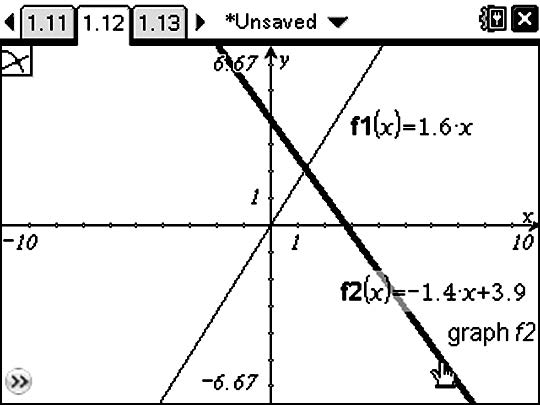•##### Download

•• ##### Device
•TI-Nspire™ CX/CX II
•TI-Nspire™ CX CAS/CX II CAS
• TI-Nspire™
• TI-Nspire™ CAS
• ##### Software

TI-Nspire™
TI-Nspire™ CAS

3.0

# Algebra I: Solving a Pair of Linear Equations by Graphing

by Brendan Kelly#### Objectives

• Graph a pair of linear equations and estimate their solution using the Intersection Point(s) command
• Verify the solution of a linear system by substitution of the solution into both equations

#### About the Lesson

The term "intersection" is familiar to all students as it applies to the intersection of two streets. The intersection of the I-95 and 407 Highways in Florida, provides a concrete model for the intersection of two lines. Both highways are close approximations to straight lines near their point of intersection, so we are able to represent them by linear equations in a coordinate system.
In this context, we can talk about the equation of a I-95 as the relationship that is satisfied only by points on highway I-95. Similarly the equation of Highway 407 is the mathematical equation satisfied only by points (or locations) on Highway 407. The intersection of these two highways is the only point that lies on both highways and therefore, satisfies both equations.

© Brendan Kelly Publishing, Inc. Reprinted with permission. www.brendankellypublishing.com